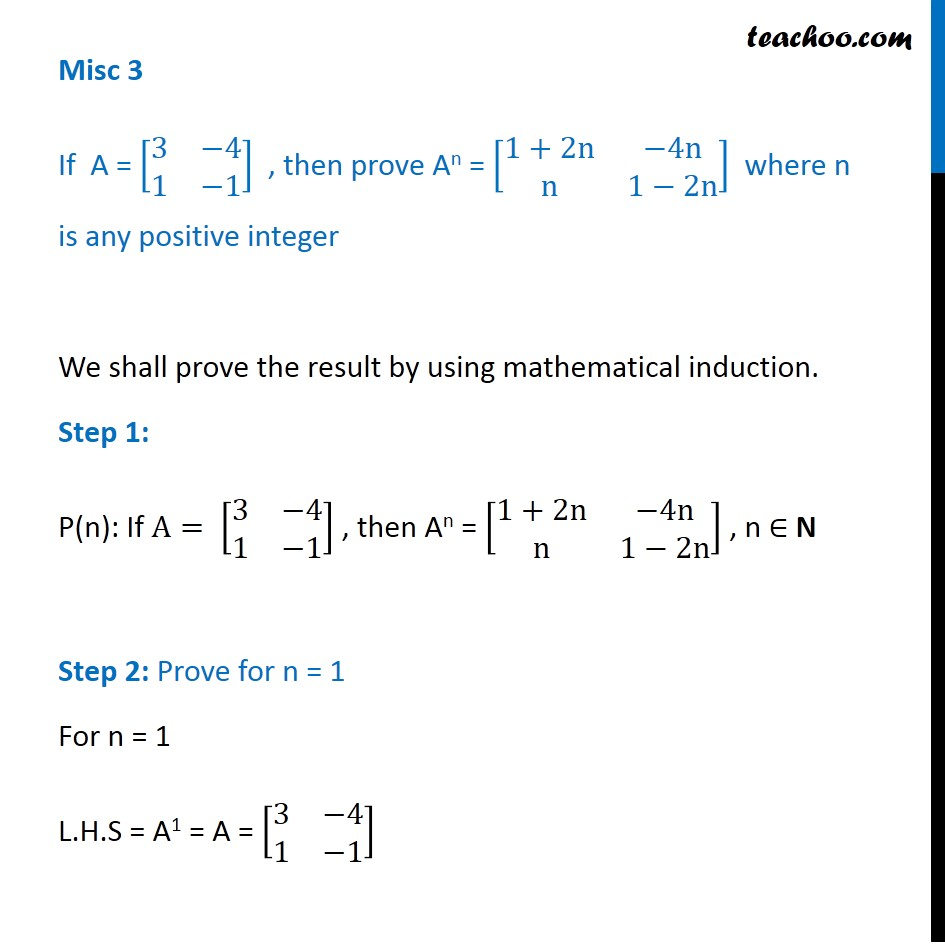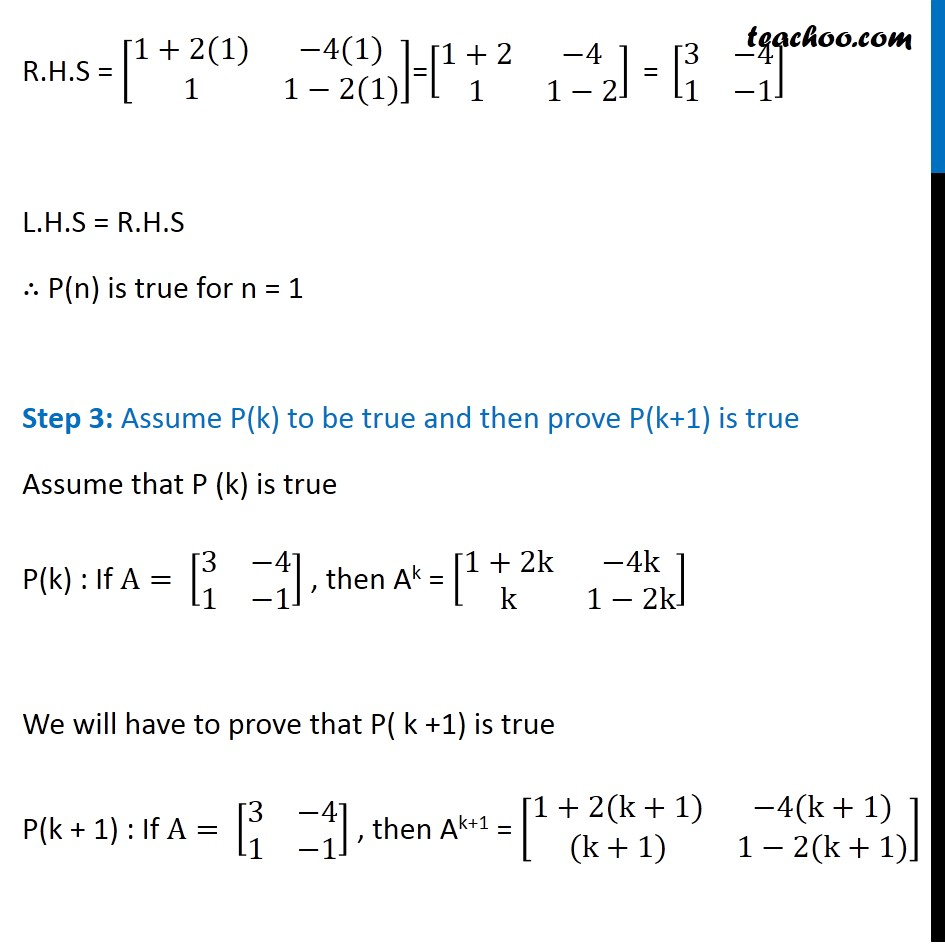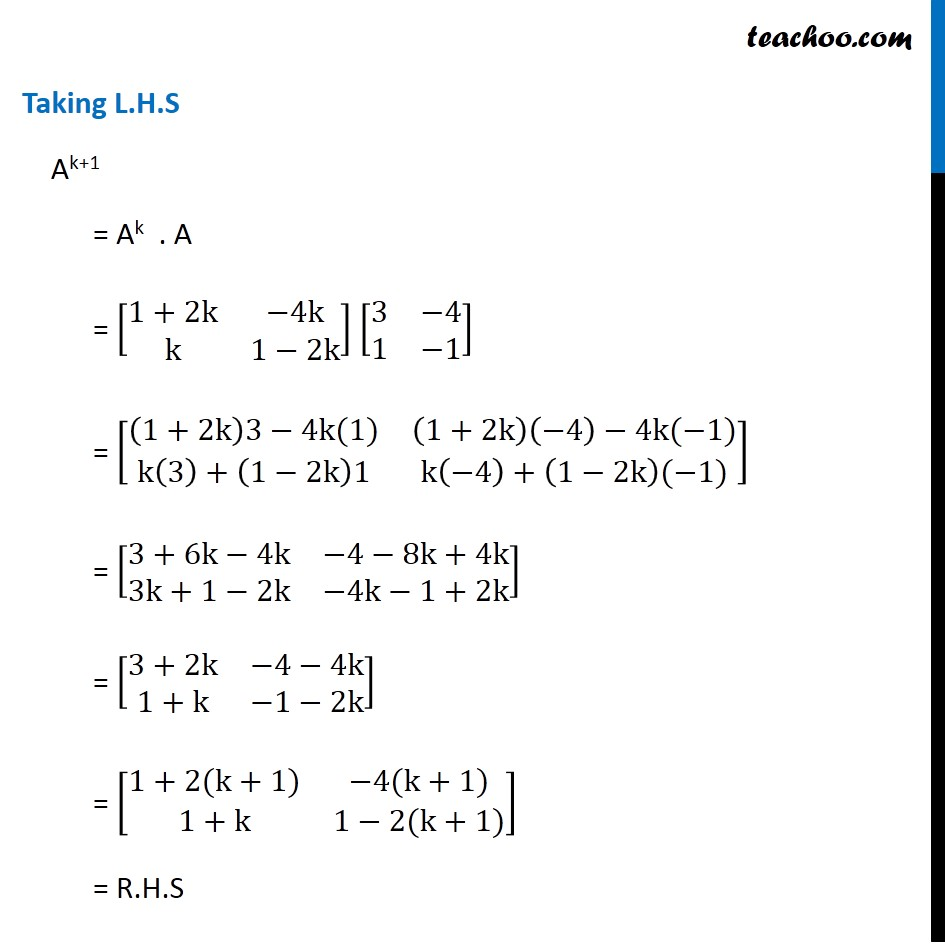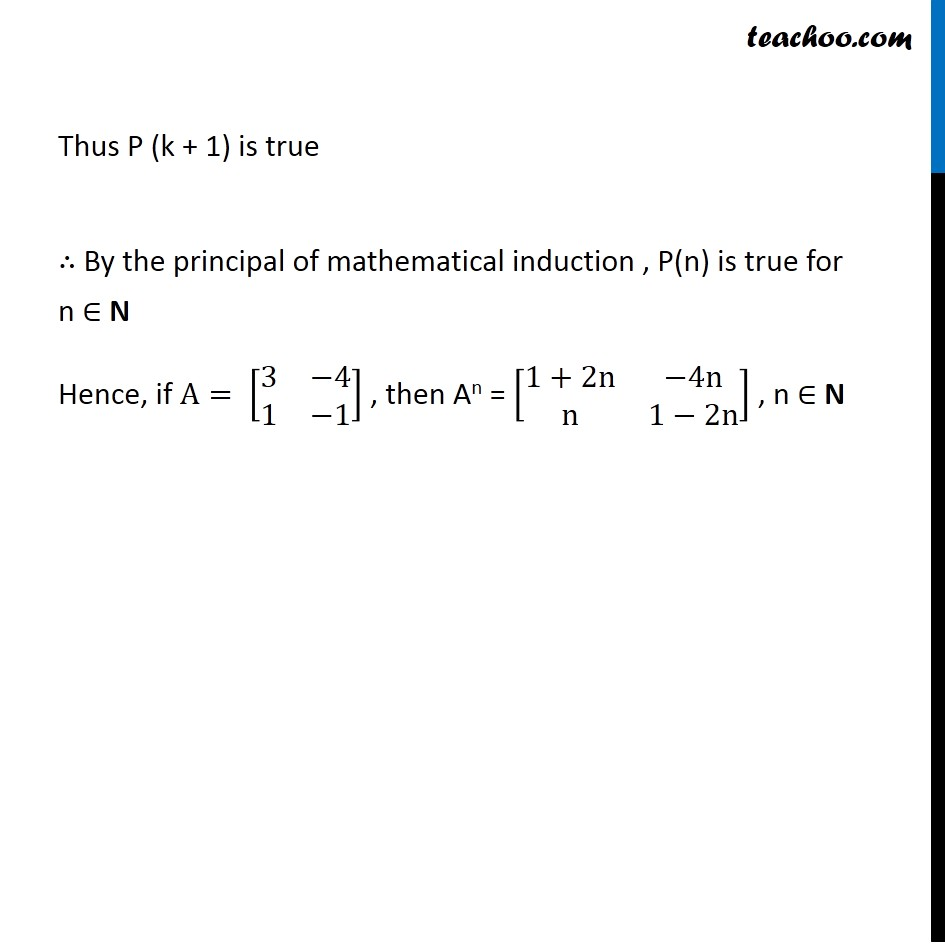Learn All Concepts of Chapter 3 Class 12 Matrices - FREE. Check - Matrices Class 12 - Full video1. Chapter 3 Class 12 Matrices
2. Concept wise
3. Proof using mathematical induction

Transcript

Misc 3 If A = [■8(3&−4@1&−1)] , then prove An = [■8(1+2n&−4n@n&1−2n)] where n is any positive integer We shall prove the result by using mathematical induction. Step 1: P(n): If A= [■8(3&−4@1&−1)] , then An = [■8(1+2n&−4n@n&1−2n)] , n ∈ N Step 2: Prove for n = 1 For n = 1 L.H.S = A1 = A = [■8(3&−4@1&−1)] R.H.S = [■8(1+2(1)&−4(1)@1&1−2(1))]=[■8(1+2&−4@1&1−2)]" = " [■8(3&−4@1&−1)] L.H.S = R.H.S ∴ P(n) is true for n = 1 Step 3: Assume P(k) to be true and then prove P(k+1) is true Assume that P (k) is true P(k) : If A= [■8(3&−4@1&−1)] , then Ak = [■8(1+2k&−4k@k&1−2k)] We will have to prove that P( k +1) is true P(k + 1) : If A= [■8(3&−4@1&−1)] , then Ak+1 = [■8(1+2(k+1)&−4(k+1)@(k+1)&1−2(k+1))] Taking L.H.S Ak+1 = Ak . A = [■8(1+2k&−4k@k&1−2k)] [■8(3&−4@1&−1)] = [■8((1+2k)3−4k(1)&(1+2k)(−4)−4k(−1)@k(3)+(1−2k)1&k(−4)+(1−2k)(−1))] = [■8(3+6k−4k&−4−8k+4k@3k+1−2k&−4k−1+2k)] = [■8(3+2k&−4−4k@1+k&−1−2k)] = [■8(1+2(k+1)&−4(k+1)@1+k&1−2(k+1))] = R.H.S Thus P (k + 1) is true ∴ By the principal of mathematical induction , P(n) is true for n ∈ N Hence, if A= [■8(3&−4@1&−1)] , then An = [■8(1+2n&−4n@n&1−2n)] , n ∈ N

Proof using mathematical induction